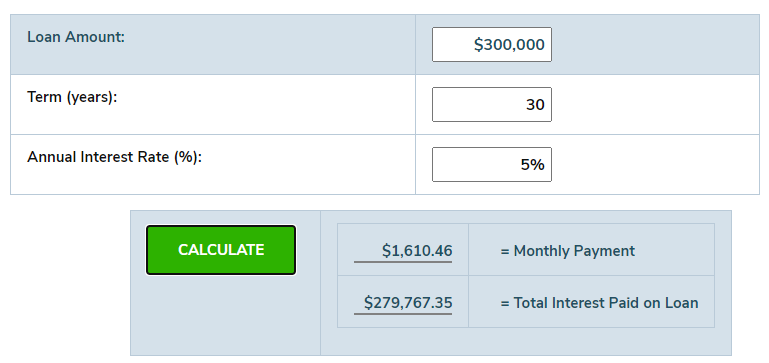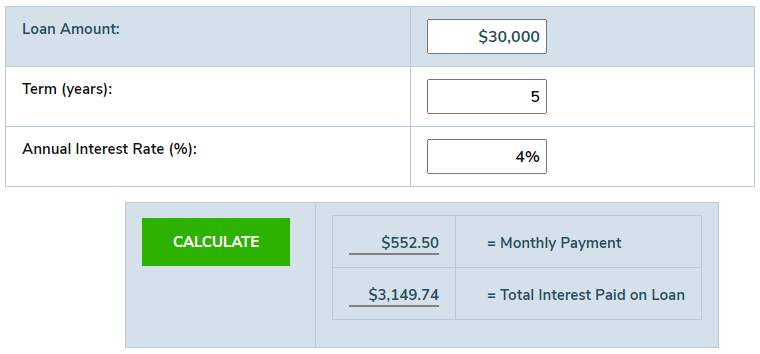## How to Use Our Loan Interest Calculator

There are three main components when determining your total loan interest:

1. Total Loan Amount. This is the total amount you are borrowing. This does not include any down payment you are making.

2. Loan Term (in years). This is the total length of the loan. Our calculator uses years to calculate the total interest accrued over this timeline.

3. Interest Rate. This is the rate charged on the loan. This should be a fixed interest rate.

To use the calculator, you will input these numbers into each section, select “CALCULATE,” and it will show your estimated monthly payment, as well as the total interest paid over the life of the loan.

• Note: This calculator is designed for fixed-rate, simple interest loans only.

## How Much Interest Will I Pay On My Mortgage? (Loan Interest Calculator Example)

If you’re looking to buy a home, you can use this calculator to determine how much interest you will pay on your mortgage over time. In the example below, we’ll look at a 30-year mortgage for \$300,000, with a fixed interest rate of 5.0%.

1. Total Loan Amount: \$300,000

2. Loan Term (in Years): 30 years

3. Interest Rate: 5.0%

Assuming you pay off the mortgage over the full 30 years, you will pay a total of \$279,767.35 in interest over the life of the loan. That is almost the original loan amount!If we compare that to a 4.0% interest rate, the total interest paid would be \$215,608.52. That is over \$60,000 of a difference in total interest, so it definitely pays to shop around for the best interest rate on your mortgage.

## How Much Interest Will I Pay On My Car Loan? (Loan Interest Calculator Example

When shopping for a car, you can use this calculator to determine how much interest you will pay on an auto loan. In the example below, we’ll look at a five-year car loan for \$30,000, with a fixed interest rate of 6.0%.

1. Total Loan Amount: \$30,000

2. Loan Term (in Years): Five years

3. Interest Rate: 6.0%

After 5 years, you will pay a total of \$4,799.04 in interest on your car loan. If you are able to shop around for a better car loan rate, you can save some money. Let’s say you find an auto loan with a 4.0% interest rate, the total interest paid on a five-year loan would be \$3,149.74. That is over \$1,500 in savings by simply finding a better rate.

Don’t Miss: How to Get Pre-Approved for an Auto Loan## How Much Interest am I Paying?

While it may be tempting to only look at the monthly payment when searching for a loan, it’s important to calculate the total amount you are actually paying over the life of the loan. On average, the longer the loan term, the more you will pay in interest.

In the mortgage example above, over 30 years, the \$300,000 mortgage will cost nearly \$600,000 total, which nearly doubles the original cost of the loan. With the auto loan example, a \$30,000 loan will end up costing nearly \$35,000 after five years.

Understanding what you will pay in total for your loan can help you determine if your purchase is worth it. While buying a home that goes up in value long-term may be worth the interest paid, buying a new car that goes down in value may end up costing you even more in the long run.

## SUMMARY

Getting the right loan for your financial situation requires knowing all the costs involved. Calculating the total interest paid on your loan will show you the true cost of borrowing money, and help you find the right loan for your goals.
For more finding the best loan rates, you can learn more in our complete guide to finding the best loans.